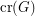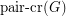# pair-crossing number

## Are different notions of the crossing number the same? ★★★

Author(s): Pach; Tóth

Problem   Does the following equality hold for every graph?The crossing numberof a graphis the minimum number of edge crossings in any drawing ofin the plane. In the pairwise crossing number, we minimize the number of pairs of edges that cross.

Keywords: crossing number; pair-crossing number